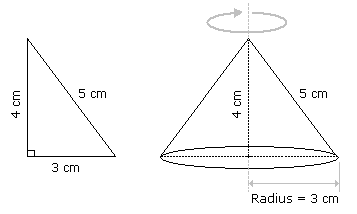## Lakshya Education MCQs

Question:

66 cubic centimetres of silver is drawn into a wire 1 mm in diameter. The length of the wire in metres will be:

Options:
 A. 84 B. 90 C. 168 D. 336

Let the length of the wire be h.

Radius = $$\frac{1}{2}mm=\frac{1}{20}cm. Then,$$

$$\frac{22}{7}\times\frac{1}{20}\times\frac{1}{20}\times h= 66$$

h =  $$\left(\frac{66\times20\times20\times7}{22}\right)=8400cm=84 m.$$

Earn Reward Points by submitting Detailed Explaination for this Question

## More Questions on This Topic :

Question 1.

A hall is 15 m long and 12 m broad. If the sum of the areas of the floor and the ceiling is equal to the sum of the areas of four walls, the volume of the hall is:

Options:
1.    720
2.    900
3.    1200
4.    1800

2(15 + 12) x h = 2(15 x 12)

h =  $$\frac{180}{27}m=\frac{20}{3}m.$$

So, volume =  $$\left(15\times12\times\frac{20}{3}\right)m^{3} = 1200m^{3}$$

Question 2.

In a shower, 5 cm of rain falls. The volume of water that falls on 1.5 hectares of ground is:

Options:
1.    75 cu. m
2.    750 cu. m
3.    7500 cu. m
4.    75000 cu. m

1 hectare = 10,000 m2

So, Area = (1.5 x 10000) m2 = 15000 m2.

Depth = $$\frac{5}{100}m = \frac{1}{20}m$$

So, Volume = (Area x Depth) =  $$\left(15000\times\frac{1}{20}\right)m^{3}=750m^{3.}$$

Question 3.

A right triangle with sides 3 cm, 4 cm and 5 cm is rotated the side of 3 cm to form a cone. The volume of the cone so formed is:

Options:
1.      $$12\pi$$ cm3
2.       $$15\pi$$cm3
3.      $$16\pi$$ cm3
4.      $$20\pi$$ cm3Clearly, we have r = 3 cm and h = 4 cm

So $$Volume,= \frac{1}{3}\pi r^{2}h = \left(\frac{1}{3}\times \pi\times3^{2}\times4\right)cm^{3} =12\pi cm^{3}.$$

Question 4.

A hollow iron pipe is 21 cm long and its external diameter is 8 cm. If the thickness of the pipe is 1 cm and iron weighs 8 g/cm3, then the weight of the pipe is:

Options:
1.    3.6 kg
2.    3.696 kg
3.    36 kg
4.    36.9 kg

Volume of iron  =  $$\left(\frac{22}{7}\times\left[(4)^{2}-(3)^{2}\right]\times21 \right)cm^{3}$$

= $$\left(\frac{22}{7}\times7\times1\times21\right)cm^{3}$$

=  462 cm3.

So, Weight of iron = (462 x 8) gm = 3696 gm = 3.696 kg.

Question 5.

A boat having a length 3 m and breadth 2 m is floating on a lake. The boat sinks by 1 cm when a man gets on it. The mass of the man is:

Options:
1.    12 kg
2.    60 kg
3.    72 kg
4.    96 kg

Volume of water displaced = (3 x 2 x 0.01) m3

= (0.06 x 1000) kg

= 60 kg.

Question 6.

50 men took a dip in a water tank 40 m long and 20 m broad on a religious day. If the average displacement of water by a man is 4 m3, then the rise in the water level in the tank will be:

Options:
1.    20 cm
2.    25 cm
3.    35 cm
4.    50 cm
So, Rise in water level = $$\left(\frac{200}{40\times20}\right)m0.25m=25cm.$$